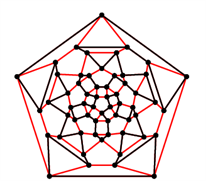# Decomposing a graph into Hamilton cyclesCollege best publication award for ‘Hamilton decompositions of regular expanders: a proof of Kelly’s conjecture for large tournaments’ (Advances in Mathemtics 237 (2013), 62-146)

Article by Professor Daniela Kühn and Professor Deryk Osthus, School of Mathematics

Graphs are very simple objects which can be used to model surprisingly complex structures. For example, this includes internet graphs, social networks, and biological networks such as the brain. Graphs can also be used to model timetabling and scheduling problems.

A graph consists of vertices, some of which are connected by edges. A fundamental problem in the field is to decide whether a graph contains a Hamilton cycle, which is a cycle containing all the vertices of the graph. This is also very difficult from an algorithmic point of view.  A far stronger requirement is to ask for a Hamilton decomposition of a graph: this is a set of Hamilton cycles decomposing all the edges of the graph - so every edge is contained in exactly one of the Hamilton cycles. For example, a Hamilton decomposition of the small rhombicosidodecahedron graph is shown above (with one cycle in red and the other in black).

One of the oldest results in Graph theory is Walecki's theorem from the 19th century that a complete graph (i.e. a graph in which there is an edge between every pair of vertices) on an odd number of vertices has a Hamilton decomposition. Hamilton decompositions are notoriously hard to obtain and so there are many open problems in this area - the most famous of these was Kelly's conjecture from 1968 which asks for an oriented version of Walecki's theorem. So every edge now has a direction and the edges in each Hamilton cycle need to be consistently oriented. This seemingly simple problem has been very resistant to attack, but was now solved for large tournaments  in the above paper. The proof spans 85 pages.

In fact, we proved a far more general result this which guarantees a Hamilton decomposition provided that a surprisingly weak structural condition holds (“robust expansion”). Such graphs arise in a variety of settings and so the result also resolves several other long-standing problems. For example, there is a surprising connection to colouring problems for graphs: we subsequently used the result to prove an old conjecture on edge-colourings of graphs, which was first posed in the 1950s (in joint work with Csaba, Lo and Treglown). Edge-colourings of graphs arise for example in timetabling problems.

Even more, our decomposition result doesn't just show that a Hamilton decomposition exists - it provides an efficient algorithm for finding it. This can be applied to the travelling salesman problem, which asks for a shortest tour visiting all vertices in a weighted graph or directed graph. The travelling salesman problem itself is NP-complete and thus probably cannot be solved efficiently by computers. But we can still ask for heuristic solutions which are guaranteed to give "good answers". Imagine putting all the possible solutions in an ordered list from lowest weight to highest weight.  Until recently, the best one could do in polynomial time for a (directed) graph on n vertices was to give an answer guaranteed not to be in the bottom (1/n)th part of the list! Our result on Hamilton decompositions can be applied to give an answer guaranteed to be in (roughly) the top half.